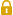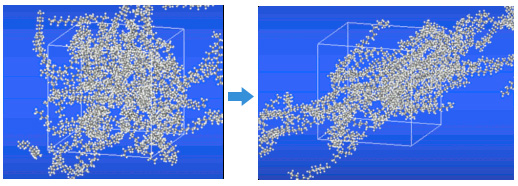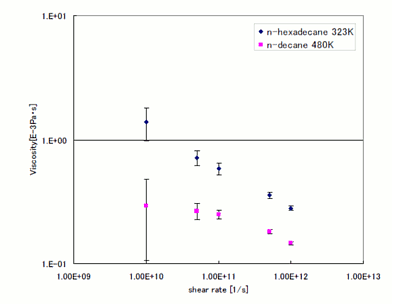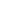Full Atomistic MD
Mechanical / Viscosity / Viscoelasticity
Materials ScienceUser Only

# Evaluation of Viscosity by Using MD

Viscosity can be evaluated by calculating the shear deformation using Lees-Edwards boundary conditions.

Results for n-decane and n-hexadecane are shown below. Using the full atomistic model created with J-OCTA, the calculations were carried out with a density of 0.613 g/cm3 and a temperature of 480 K for n-decane and a density of 0.753 g/cm3 and a temperature of 323 K for n-hexadecane. The numbers of molecules in the system for the two substances were respectively 200 and 100. The viscosity was evaluated while varying the shear rate from 1e+12 [1/s] to 1e+10 [1/s]. VSOP was used for the MD calculations (Fig. 1).

Fig. 2 shows a double logarithmic plot of the calculated viscosity versus the shear rate. It can be seen that the viscosity increases with decreasing shear rate; however, for n-decane, the viscosity approaches a constant value when the shear rate is at 1e+10 [1/s] or below.

In the viscosity evaluation using the Green-Kubo method in Reference , the viscosity of n-decane under similar conditions is 0.178 [e-3 Pa·s] and the viscosity of n-hexadecane is 1.17 [e-3 Pa·s].Fig. 1. Simulation system with the Lees-Edwards boundary condition.Fig. 2. Evaluated viscosity.

• Reference
•  S. T. Cui, P. T. CUMMINGS and H. D. COCHRAN, Molecular Physics, 1998, 93, 1, 117-121This website uses cookies to improve functionality and performance. If you continue browsing the site, you are giving implied consent to the use of cookies on this website. If you want to know more or refuse consent, read our Cookie Policy.

Accept